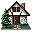Home Existing users log in here or new users sign up here. Nature of Motion Types of Motion Galileo's Pisa Experiment Projectile Motion Juggling

### Non-simultaneous drop of two objects: Testing Experiment

#### Aim

This testing experiment requires the application of your model for motion with constant acceleration developed in the Types of motion cycle and the model you have just developed regarding the depence of free fall motion on the mass of the object.

#### Prior Knowledge

• Dependence of free fall acceleration on mass.
• Graphing skills.
• Reference frames.
• Kinematics knowledge: position, velocity and acceleration.

#### Description of the Experiment

In this variation of Galileo's experiment, two balls are dropped from the same height. However, one ball is released a fraction of a second after the other.

##### Basic Prediction

Because one ball has a slight head start over the other, it has advanced a certain distance before the second ball is released. At the moment when the second ball is released, let's call the vertical distance between the two balls y. Based on your model of free fall and understanding of kinematics, predict what will happen to this distance of separation between the balls as they fall. To help make prediction, try to represent the motion of the balls in as many ways as you can - motion diagrams, graphs and mathematics.

Watch the video, take data from the video frame by frame, measuring the distance between the two balls. Then plot a graph of distance vs time. Before you do, predict what sort of curve you expect to obtain from the graph. Use your knowledge of motion with constant acceleration to aid you with your prediction.

Use hypothetico-deductive reasoning to formulate your predicition:

BEFORE EXPERIMENT AFTER EXPERIMENT
 IF both balls accelerate at the same rate, THEN the distance between them should.........
 BUT/AND the distance.........., THEREFORE ...................................

#### Questions

If you are struggling to explain what you saw for either the basic or advanced predictions, consider one of the following two approaches to explain what you observe:

1. ##### Graphical Method
• Plot graphs of velocity vs time for both balls. It is most important to choose a suitable starting time which is common to both balls. Choosing the time when the second ball is released is probably the best.

• How do you obtain the distance travelled by each ball from its velocity vs time graph?

• How then would you find the distance between the two balls from the two graphs?

• Does this explain what you saw?

2. ##### Algebraic method
• At some time t during the fall, how would you express the distance travelled by either one of the balls as a function of time?

• How would you express the difference in distance between the balls as a function of time? Be careful to define a starting time common to both balls.

• Does this explain what you see?

3. ##### Motion Diagram method
• Draw motion diagrams of the two falling objects. Place the motion diagrams side by side and pretend that the second ball is released exactly one time step unit after the first. Does this help you make a qualitative prediction?